• 因此，课本中的部分...股票基本面分析实际操作：1、数据准备利用tushare获取股票的相关数据，但因所需的数据不在同一个接口就能读取到，因此分两次获取。(ps：excel的合并没用Python)import tushare as ts #引入t...
注：tushare接口已进行更新，之前的旧接口不可用，(新接口：https://tushare.pro/)。因此，课本中的部分操作已不可用。股票基本面分析实际操作：1、数据准备利用tushare获取股票的相关数据，但因所需的数据不在同一个接口就能读取到，因此分两次获取。(ps：excel的合并没用Python)import tushare as ts                    #引入tusharets.set_token('8fd9fa6fe51e5f0a0cbd5b18b6e8ffaea51002f32a1770acb69d9466')      #执行一次在本机保存token，以后无需执行pro= ts.pro_api()          #初始化接口data= pro.stock_basic()                   #读取相关股票数据data.to_excel("上市公司基本面1.xlsx")         #把数据读入到excel里df = pro.daily_basic(ts_code='', trade_date='20201210', fields='ts_code,trade_date,total_mv,pe')                   读取total_mv,pe数据df.to_excel("上市公司基本面2.xlsx")         #把数据读入到另一个excel里2、数据类型转换从excel中读取数据时，pandas会将数据自动转换为数值类型。因此，当股票代码前两位为00时，这两个数值就会丢失。故我们应将code字段设置为字符串。(此处提及的code是股票基本数据的一个字段，相关字段见补充)df = pd.read_excel('上市公司基本面.xlsx',dtype={'code':'str'})       #读取excel表格，并把code设置字符串类型print(df)df.set_index('ts_code',inplace=True)         #将code设为索引列结果：excel截图.pngexcel截图2.png3、相关数据查看股票的基本信息print(df.shape)           #4083只股票print(df.columns)         #显示数据的相关字段a = len(df.industry.unique())    #显示行业数b = len(df.area.unique())          #显示股票归属的省份c = df.groupby('area').area.count().sort_values(ascending=False)         #按地区统计上市公司数量，体现地区经济实力print(a)print(b)print(c)结果：运行结果部分截图.png4、统计每年股票发行量并绘制折线图year = df.list_date.astype('str').str[:4]           #转换为字符串，提取年份yearnum = df.groupby(year).name.count()               #按年份统计，每年股票发行量import matplotlib.pyplot as plt                         #引入绘图库plt.rcParams['font.sans-serif']=['SimHei']                #设置中文yearnum.plot(title='年IPO数量',marker='o',fontsize=16)          #绘制图像plt.show()按年份统计股票的发行量，判断当年是牛市还是熊市结果：1990-2020年PIO数量.png5、计算市场的平均市盈率可以发现在这个excel表格中，有一些股票的pe(市盈率)是显示为0的，这是因为这些股票是亏损的，因此在计算市场平均市盈率时要剔除这一部分的数据。pe_mean = df[df.pe > 0].pe.mean()         #剔除亏损的股票后计算均值print(pe_mean)简单的算数平均.pngpe_mean2 = np.sum(df.pe * df.total_mv)/df.total_mv.sum()             #以总市值为权重求加权的peprint(pe_mean2)加权平均.png6、对每个版块进行统计df['board'] = df.index.str[:2]d = df.groupby('board').pe.agg([('pe均值','mean'),('股票数','count')])print(d)结果：分版块统计结果.png附：名称         类型               描述ts_code str           TS代码symbol  str           股票代码name    str           股票名称area             str              所在地域industry    str              所属行业list_date   str 上市日期price   float   Y   发行价格pe  float   市盈率(总市值/净利润， 亏损的PE为空)total_mv    float   总市值 (万元)更多的字段可前往Tushare官网查询：https://waditu.com/
展开全文• 一、初识PandasPandas 是基于 NumPy 的一个非常好用的库，它有两种自己独有的基本数据结构Series (一维)和 DataFrame(二维)，它们让数据操作更简单了。虽然Pandas有着两种数据结构，但它依然是 Python 的一个库，...
一、初识PandasPandas 是基于 NumPy 的一个非常好用的库，它有两种自己独有的基本数据结构Series (一维)和 DataFrame(二维)，它们让数据操作更简单了。虽然Pandas有着两种数据结构，但它依然是 Python 的一个库，所以，Python 中有的数据类型在这里依然适用，也同样还可以使用类自己定义数据类型。在金融数据分析领域，Pandas更是起到了非常重要的作用，比如用于量化交易。Pandas 纳入了大量库和一些标准的数据模型，提供了高效地操作大型数据集所需的工具，对于亿级的数据处理也如鱼得水。二、Pandas基本操作1、Series的创建Series的创建主要有三种方式：1)通过一维数组创建Seriesimport numpy as npimport pandas as pd#创建一维数组a = np.arange(10)print(a)s = pd.Series(a)print(s)输出如下：2)通过字典的方式创建Seriesimport numpy as npimport pandas as pd#创建字典d = {'a':1,'b':2,'c':3,'d':4,'e':5}print(d)s = pd.Series(d)print(s)输出如下：3)通过DataFrame中的某一行或某一列创建Series参考下面DataFrame第三种创建方式中的s = df3[‘one’]。2、DataFrame的创建DataFrame的创建主要有三种方式：1)通过二维数组创建DataFrameimport numpy as npimport pandas as pd#创建二维数组a = np.array(np.arange(12)).reshape(3,4)print(a)df1 = pd.DataFrame(a)print(df1)输出如下：2)通过字典的方式创建DataFrame以下以两种字典来创建数据框，一个是字典列表，一个是嵌套字典。import numpy as npimport pandas as pdd1 = {'a':[1,2,3,4],'b':[5,6,7,8],'c':[9,10,11,12],'d':[14,14,15,16]}print(d1)df1 = pd.DataFrame(d1)print(df1)d2 = {'one':{'a':1,'b':2,'c':3,'d':4},'two':{'a':5,'b':6,'c':7,'d':8},'three':{'a':9,'b':10,'c':11,'d':12}}print(d2)df2 = pd.DataFrame(d2)print(df2)输出如下：3)通过DataFrame的方式创建DataFrame我们取出2)中的df2来创建df3df2 = pd.DataFrame(d2)print(df2)df3 = df2[['one','two']]print(df3)s = df3['one']print(s)输出如下：三、处理股票数据接下来，我们通过实例来学习Pandas在处理股票数据上的应用。我们使用pandas_datareader来获取阿里巴巴的股票数据。1)导入以下库：import pandas as pdimport pandas_datareader.data as web#绘图使用import matplotlib.pyplot as plt#获取时间使用import datetime2)设置股票名称和时间参数name = "BABA"start = datetime.datetime(2015,1,1)end = datetime.date.today()3)获取股票数据prices = web.DataReader(name, "google", start, end)4)查看prices的类型print(type(prices))打印如下：可以看到返回的数据类型就是DataFrame类型。5)查看股票的摘要信息print(prices.describe()打印 如下：Open        High         Low       Close        Volumecount  791.000000  791.000000  792.000000  792.000000  7.920000e+02mean   106.632099  107.793186  105.355164  106.614520  1.610571e+07std     38.191772   38.539981   37.719848   38.156416  9.941683e+06min     57.300000   58.650000   57.200000   57.390000  2.457439e+0625%     79.855000   80.945000   79.157500   79.935000  1.003487e+0750%     91.000000   91.740000   89.925000   90.705000  1.350020e+0775%    119.315000  120.400000  118.462500  120.205000  1.879724e+07max    204.830000  206.200000  202.800000  205.220000  9.704593e+07再来打印最新的三条信息print(prices.tail(3))Open    High     Low   Close    VolumeDate2018-02-21  189.37  193.17  188.46  188.82  220715852018-02-22  190.20  190.74  187.77  188.75  122828432018-02-23  190.18  193.40  189.95  193.29  169372756)绘图我们将阿里巴巴的股票数据按照开盘价绘图。plt.plot(prices.index, prices["Open"])plt.show()从图中我们可以看到阿里巴巴的股票一路攀升，细心点发现每年的11月都有一个高点。四、总结Pandas是以NumPy和Matplotlib为基础封装的金融数据分析的库，对于量化交易十分有用，通过可视化的效果能帮我们一定程度分析股市的走向。
展开全文• 对模型做一阶差分，希望得到一个平稳的时间序列 一阶差分后，模型基本平稳： p=ts.adfuller(strike_info['close']) #print p price_log=strike_info['close'].diff() AR(p)模型，PACF会在lag=p时截尾，也就是，...
选择期货CFFEX.IF1808，截止到当日的1000条收盘价格走势：# encoding: utf-8import talibfrom talib.abstract import SMAimport numpy as npimport pandas as pdimport mathimport datetimefrom collections import dequefrom gm.api import *  #掘金import matplotlib.pyplot as pltimport matplotlib as mplimport mpl_finance as mpfimport matplotlib.dates as mpdimport seaborn as snsimport statsmodels.tsa.stattools as tsimport statsmodels.api as smfrom statsmodels.tsa.arima_model import ARMAfrom scipy import  statsfrom statsmodels.graphics.api import qqplotset_token('****************************') #自行填写自己的tokennow=datetime.datetime.now().date()last_day=get_previous_trading_date(exchange='SHSE',date=now)index_futures=get_continuous_contracts(csymbol='CFFEX.IF',start_date=last_day,end_date=last_day)#print index_futuresstrike_info=history_n(symbol='CFFEX.IF1808',frequency='60s',end_time='2018-07-01',fields='symbol,close,frequency,cum_volume',count=1000,df=True)strike_info.dropna()price=np.array(strike_info['close'])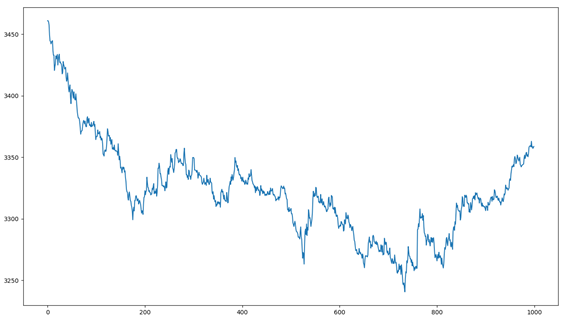一个时间序列，他可能是有趋势的，是不平稳的，所以如果不平稳需要做差分。ADF检测结果：95%置信区间，p=0.0076，99%置信区间下，p=-3.5。对模型做一阶差分，希望得到一个平稳的时间序列一阶差分后，模型基本平稳：p=ts.adfuller(strike_info['close'])#print pprice_log=strike_info['close'].diff()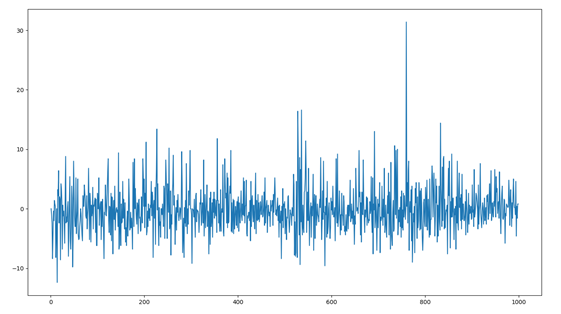AR(p)模型，PACF会在lag=p时截尾，也就是，PACF图中的值落入宽带区域中。MA(q)模型，ACF会在lag=q时截尾，同理，ACF图中的值落入宽带区域中。用ACF(自相关系数)或者PACF(偏自相关系数)观察模型：fig = plt.figure(figsize=(12,8))ax1=fig.add_subplot(211)fig = sm.graphics.tsa.plot_acf(strike_info['close'],lags=40,ax=ax1)ax2 = fig.add_subplot(212)fig = sm.graphics.tsa.plot_pacf(strike_info['close'],lags=40,ax=ax2)plt.show()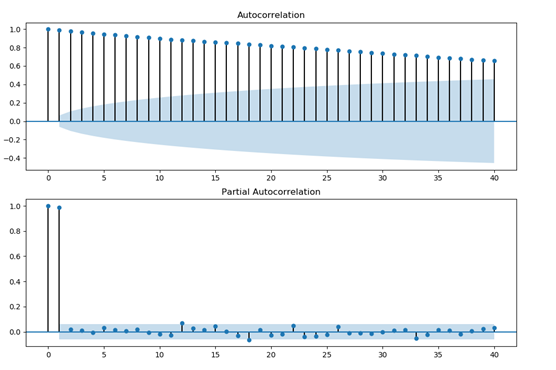优先选择PACF图，因为PACF大约在lag=1时截尾，即PACF的值落入宽带区域中选择AR(P=1)的模型进行自回归拟合，得到拟合效果：arma_mod80 = sm.tsa.ARMA(strike_info['close'],(1,0)).fit()print(arma_mod80.aic,arma_mod80.bic,arma_mod80.hqic)resid = arma_mod80.residprint(sm.stats.durbin_watson(arma_mod80.resid.values))print(stats.normaltest(resid))fig = plt.figure(figsize=(12,8))ax = fig.add_subplot(111)fig = qqplot(resid, line='q', ax=ax, fit=True)plt.show()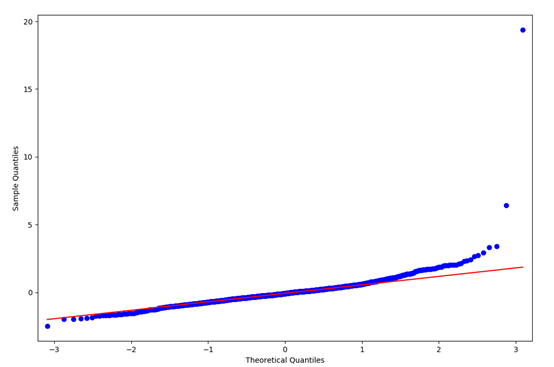检验：计算得到序列的残差，基本为白噪音fig = plt.figure(figsize=(12,8))ax1 = fig.add_subplot(211)fig = sm.graphics.tsa.plot_acf(resid.values.squeeze(), lags=40, ax=ax1)ax2 = fig.add_subplot(212)fig = sm.graphics.tsa.plot_pacf(resid, lags=40, ax=ax2)plt.show()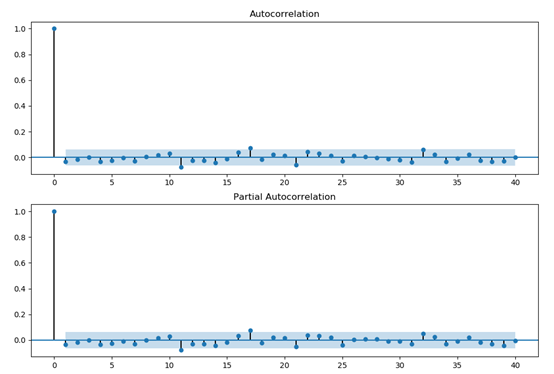用自回归拟合的模型进行预测，结果如下：fig=plt.figure(figsize=(15,7))price2=strike_info=history_n(symbol='CFFEX.IF1808',frequency='60s',end_time='2018-07-01',fields='symbol,close,frequency,cum_volume',count=1000,df=True)['close']price3=strike_info=history_n(symbol='CFFEX.IF1808',frequency='60s',end_time=now,fields='symbol,close,frequency,cum_volume',count=1000,df=True)['close']print len(price2)fit = arma_mod80.predict(0, 1100)plt.plot(range(1100),fit[:1100],label='predict')plt.plot(price2,label='price')plt.legend(loc=4)plt.show()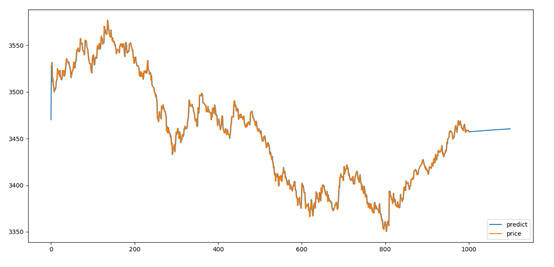展开全文• 一、砖型图简介。在所有行情软件中，记录价格波动轨迹的几乎都是K线图，只有在很少的程序化量化软件中才有砖型图的身影。热卷hc2005砖型图顾名思义，相当于是用砖头一块一块地拼凑而成记录价格波动轨迹的图形，称之...
一、砖型图简介。在所有行情软件中，记录价格波动轨迹的几乎都是K线图，只有在很少的程序化量化软件中才有砖型图的身影。热卷hc2005砖型图顾名思义，相当于是用砖头一块一块地拼凑而成记录价格波动轨迹的图形，称之为“砖型图”。每一块砖的尺寸都一致，这里的尺寸是指行情每上涨或下跌一定距离，就绘制下一块砖型图，如果行情没有达到这个尺寸，无论行情走了多久，我们都不会更新砖型图。因此，砖型图的走势始终就只有上涨和下跌两个方向。二、砖型图的优势。(一)让行情走势结构更加的清晰。在k线图中，行情如果遇到小幅的盘整，行情在一个很小的区间内来回突突，迷惑你，并且看上去走了很长的一段时间，实际上它并没有上涨或下跌多少。但是，采用砖型图看行情的话，如果将这个区间设置成砖型图的尺寸，那么在这个区间只绘制一块砖，从而减少交易者在此区间的错误判断。tick行情走势尺寸=2，砖型图(二)降低周期参数对整个公式或指标计算的影响。我们都知道，k线是按照时间周期来进行绘制的，因此每到一个时间节点它都会进行切换并重新绘制，这样的话如果在横盘期间价格一直不怎么动，指标一样要滚动计算。拿均线来说，这样计算出来的值很可能就是一根横向整理的线条，但如果采用砖型图进行绘制均线或计算其他指标的话，就会降低这样的影响程度。如下图所示：一旦采用砖型图，就没有时间概念了，只有空间的概念。三、砖型图代码编写。砖型图我们可以也看做没有影线的k线，砖的最顶部我称作最高价，最底部我称为最低价。绘制砖型图之前，首先我们要了解单块砖型图的构造和多块砖型图如何组合。假设，下列行情的开盘价为3555。(一)单块砖型图。假设，上图是开盘前5分钟的走势，开盘下跌。当价格下跌到5跳的时候，第一块砖的最高价就是开盘价，最低价就是开盘价-尺寸(5跳)，如果开盘价=3555。砖的最高价就是3555砖最低价就是3555-5=3550。如果开盘后上涨了5跳，那么砖最低价就是开盘价3555，最高价就是3560。这是第一块砖型图的画法。但是，最重要的是组合砖型图的画法，组合方式只有4种。(二)组合砖型图画法。1.上涨行情的组合。如果市场一开盘就往上走并且超过了2个尺寸，也就是10跳。当第一块绘制完成之后，价格回调并没有达到5跳，进而继续上扬上涨了5跳，那此时砖型图的最高最低价如何确定。最高价：上一块的最高价+5跳 ，即：3560+5 = 3565。最低价：上一块的最高价，即：3565。如果行情继续往上，同时回调不够5跳，那么继续按照此逻辑绘制。2.下跌行情组合。如果市场一开盘就往下走并且超过了2个尺寸，也就是10跳。当第一块绘制完成之后，价格回调并没有达到5跳，进而继续下跌了5跳，那此时砖型图的最高最低价如何确定。开盘价：3555最高价：上一块的最低价 ，即：3550。最低价：上一块的最低价-5跳，即：3550-5 = 3545。如果行情继续往下，同时回调不够5跳，那么继续按照此逻辑绘制。3.上涨后反转组合。当市场已经走了两块砖了，此时遇到阻力位掉头并向下跌了5跳，此时的最高价最低价如何确定。最高价：上一块的最低价 ，即：3560。最低价：上一块的最低价-5跳，即：3560-5 = 3555。最低价又回到开盘价位置。如果行情继续往下，同时回调不够5跳，那么继续按照此逻辑绘制。4.下跌后反转组合。当市场已经走了两块砖了，此时遇到支撑位掉头并向上涨了5跳，此时的最高价最低价如何确定。最高价：上一块的最高价+5跳 ，即：3550+5 = 3555。最低价：上一块的最高价，即：3550。最高价又回到开盘价位置。如果行情继续往上，同时回调不够5跳，那么继续按照此逻辑绘制。5.震荡反转组合。如果出现以下行情，绘制方法是和上面的三种绘制方法是一致的。主要出现在行情在某个区间内上涨5跳后突然反转下跌5跳，反转结束之后紧接着有反方向涨5跳，如此反复触发设定的尺寸。6.一个tick多次触发多个尺寸组合。这种情况，是比较少见的，比如一笔超级大单子突然出现，将行情一次就推了100跳，那么此时应该怎么绘制。如果出现这种情况，用100/5=20个尺寸，直接循环绘制20次，最高价和最低价按照上面4个方法进行绘制，下跌100跳也是按照这样的思路。(三)代码实现。1.总的绘制逻辑：(1)计算最新一个tick与上一个tick的差值，然后除以尺寸，得出需要绘制的次数。(2)当出现余数时，当前这块砖绘制完成后，将余数传回下一个tick继续累加。(3)绘制完一块时，通过count = count +1 的方式作为砖型图的x轴，y轴是最高价和最低价。2.代码绘制逻辑。(1)导入模块并获取8000个tick数据，品种使用rb2005。import pandas as pdfrom tqsdk import TqApifrom datetime import timedeltafrom matplotlib.path import Pathfrom matplotlib.patches import PathPatchimport matplotlib.pyplot as pltimport numpy as npapi = TqApi()ticks = api.get_tick_serial("SHFE.rb2005",8000)(2)首先我们要计算最新一个tick与上一个tick的差值，然后除以尺寸，得出需要绘制的次数和需要传回继续累加的余数。参数变量设置def renko_charts():dis_sum = 0       # 每更新一个tick都将涨跌幅累加，直到至少满5跳绘制后，将余数传回继续累加dis_sum_list = [] # 涨跌幅累加一次，就将当时的累计涨跌幅存入，方便后续判断上一根是否亏损。Renko_h = []      # 记录每块砖的最高价，用于计算均线。location = []     # 存储每一块砖的坐标，后面绘制。dot = 5           # 砖的尺寸count = 0         # X轴坐标k_list = []       # 用于存储砖的最高价最低价如果变动了至少1个尺寸，计算绘制次数和余数。decimals 代表余数(需要传回继续累加)，integer代表整数(需要绘制的次数)for i in range(len(ticks)):last_price = ticks['last_price'].iloc[i]last_time  = pd.to_datetime(ticks.datetime.iloc[i]) + timedelta(hours=8)if i ==0:k_list.append(last_price)Renko_h.append(last_price)else:"统计换线阀值 >= 5，换线"dis_sum = dis_sum + (last_price - ticks['last_price'].iloc[i-1])"如果变动了5个点，就换线"if abs(dis_sum) >= dot:"计算循环绘制次数-阀值的倍数，下一个Tick继续累加"if dis_sum / dot<0:decimals = float('-0.' + str(dis_sum / dot).split('.'))else:decimals = float('0.' + str(dis_sum / dot).split('.'))integer = int(float(str(abs(dis_sum) / dot).split('.')))首先我们要计算出第一块是阴还是阳，然后在根据前一块的阴阳和当前阴阳情况进行绘制最新的一块砖。绘制第一块：也就是说只判断第一次统计等于或超过尺寸的情况。"循环绘制-连续出现N条砖"for j in range(integer):dis_sum_list.append(dis_sum)count += 1print('---------------------------------------------------------')print('---------------------------------------------------------')"如果是上涨"if len(Renko_h) == 1:# print('换线了---------------')if dis_sum_list[-1] > 0:k = k_list[-1] + dot"最低价"k_list.append(k_list[-1])"最高价"k_list.append(k)dis_sum = decimals  # 将剩余部分传回最新一个tick继续累加涨跌幅print("最高价：%s,最低价：%s"%(k_list[-1],k_list[-2]))"基准价"Renko_h.append(k)print(Renko_h)"砖形图坐标"location.append([(count-1,k_list[-2]) ,(count-1,k_list[-1]),(count,k_list[-1]),(count,k_list[-2]), (count, last_price)])elif dis_sum_list[-1] < 0:# print('换线了---------------')k = k_list[-1] - dot"最低价"k_list.append(k)"最高价"k_list.append(k_list[-1])dis_sum = decimals  # 将剩余部分传回最新一个tick继续累加涨跌幅print("最高价：%s,最低价：%s" % (k_list[-1], k_list[-2]))Renko_h.append(k_list)location.append([(count-1,k_list[-2]) ,(count-1,k_list[-1]),(count,k_list[-1]),(count,k_list[-2]), (count, last_price)])绘制第N块：在上面代码中，我们已经得到了第一块的最高价最低价，以及坐标。最高价：k_list[-1]最低价：k_list[-2]那么在接下来我们，我们绘制第N块砖。当上一块是阳，绘制当前阴阳两种情况。当上一块是阴，绘制当前阴阳两种情况的思路和上述一致。绘制砖型图到画布：rb2005合约tick数据，尺寸 = 2跳。length=10average = []for i in range(len(Renko_h)):if iaverage.append(np.nan)else:average.append(np.mean(Renko_h[i - length:i]))print(average)fig, (ax1,ax2) = plt.subplots(2,1,figsize = (20,10))codes = [Path.MOVETO] + [Path.LINETO] * 3 + [Path.CLOSEPOLY]for i in range(len(location)):if i==0:if location[i] > 0 and dis_sum_list>0:path = Path(location[i], codes)pathpatch = PathPatch(path, facecolor='r', edgecolor='black')ax2.add_patch(pathpatch)ax2.autoscale_view()elif location[i] < 0 and dis_sum_list<0:path = Path(location[i], codes)pathpatch = PathPatch(path, facecolor='g', edgecolor='black')ax2.add_patch(pathpatch)ax2.autoscale_view()else:if location[i] > location[i-1]:path = Path(location[i], codes)pathpatch = PathPatch(path, facecolor='r', edgecolor='black')ax2.add_patch(pathpatch)ax2.autoscale_view()else:path = Path(location[i], codes)pathpatch = PathPatch(path, facecolor='g', edgecolor='black')ax2.add_patch(pathpatch)ax2.autoscale_view()ax2.plot(average)ax1.plot(ticks['last_price'])plt.show()if __name__ == '__main__':renko_charts()run:rb2005合约tick数据，尺寸 = 2跳。尺寸 = 3跳。尺寸 =4跳。尺寸 = 5跳。6.上面的不同尺寸的砖型图中，我们可以得出，尺寸越大砖的数量就越少。反之，越多。总结。本文主要分享了砖型图的简介，算法和代码实现。利用砖型图进行交易的人非常少，但并不意味着用它赚不了钱，因为大部分的行情软件都只有按时间节点来绘制的k线图，并没有按照空间来进行绘制。其实k线图和砖型图的最大区别是，一个是按照时间绘制，一个是按照空间来绘制，两者的出发点悬殊非常大，思考的方式也不一样。当然还有另外2种描述价格的算法，量能为阀值的，量能k线，以包含关系的，缠论，这些都值得去研究一下。
展开全文• 问：如何使用Python分析大数据毫不夸张地说，大数据已经成为任何商业交流中不可或缺的一部分。桌面和移动搜索向全世界的营销人员和公司以空前的规模提供着数据，并且随着物联网的到来，大量用以消费的数据还会呈指数...
• 最近在研究一些股票的东西，想批量获取所有沪深股票基本面的数据，然后就上网搜索到一个非常好的股票python接口包Tushare，而且使用方法超级简单，很适合我这种python初学者菜鸟。1.首先需要先下载这个接口包，我是...
• 利用Python进行量化分析，AkShare获取股票基本面财务数据。进行基本面数据分析，pe市盈率、ps市销率、pb市净率、总市值等数理统计，以及图表展示。基于莫伦卡选股模型进行编码，对A股300支股票进行模型运行，得到选...
• 利用Python进行量化分析，AkShare获取股票基本面财务数据。进行基本面数据分析，pe市盈率、ps市销率、pb市净率、总市值等数理统计，以及图表展示。基于莫伦卡选股模型进行编码，对A股300支股票进行模型运行，得到选...
• """利用python进行股票技术分析--以茅台为例通过下述策略对利用python进行股票技术分析，可以举一反三，并且能够实现复杂的技术分析策略，同时可以同python的宏观数据、公司基本面数据、舆情等分析结合起来。...
• 通过下述策略对利用python进行股票技术分析，可以举一反三，并且能够实现复杂的技术分析策略，同时可以同python的宏观数据、公司基本面数据、舆情等分析结合起来。 策略原理：在股票下跌过程中，当出现上涨锤子线...大数据 算法 数据挖掘 机器学习
• 泰坦尼克号数据集分析 使用Seaborn库中包含的titanic数据集进行一些数据统计。 Seaborn是一个图形库，Anaconda已包含此库。数据集参见： https://github.com/mwaskom/seaborn-data import seaborn as sns tit = sns...
• 基本面因子价值因子盈利脑子成长因子资本结构因子运营因子流通性因子2.技术因子动量因子趋势因子市值因子波动因子成交量因子按照因子来源的角度如下：公司层面：价值因子成长因子规模因子等市场层面：趋......

# python股票基本面分析python 订阅